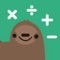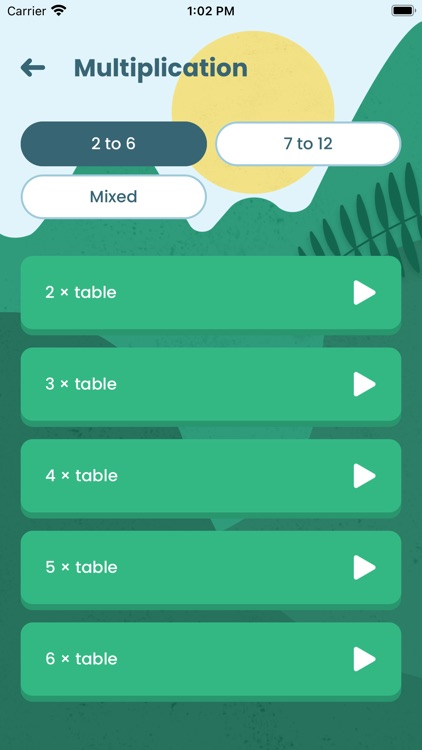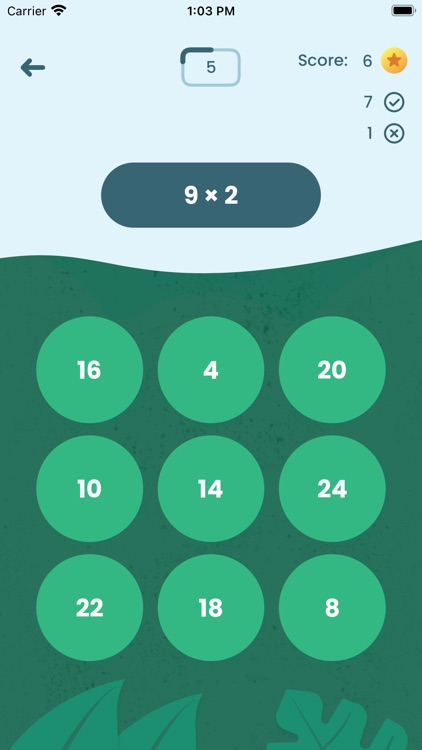## Bash Maths has been developed by teachers to provide a fun way for students to practise quick recall of essential number facts# Bash Maths

by Bash MathsBash Maths has been developed by teachers to provide a fun way for students to practise quick recall of essential number facts. We have carefully designed Bash Maths to help learners make accelerated progress across a range of content from the Mathematics curriculum for children in Key Stage 1 and Key Stage 2.### App Details

Version
2.0.0
Rating
NA
Size
12Mb
Genre
Education Family Puzzle
Last updated
October 16, 2020
Release date
September 20, 2020

### App Screenshots### App Store Description

Bash Maths has been developed by teachers to provide a fun way for students to practise quick recall of essential number facts. We have carefully designed Bash Maths to help learners make accelerated progress across a range of content from the Mathematics curriculum for children in Key Stage 1 and Key Stage 2.

The more students play, the more confident they become with number.

The Bash Maths app will support students to cover a huge range of mathematics curriculum objectives and make accelerated progress towards their learning goals at home and in school.

Curriculum coverage

Key Stage 1:

• interpret mathematical statements involving addition (+), subtraction (−) and equals (=) signs

• represent and use number bonds and related subtraction facts within 20

• add and subtract one-digit and two-digit numbers to 20, including 0

• count in steps of 2, 3, and 5 from 0, and in 10s from any number, forward and backward

• recall and use addition and subtraction facts to 20 fluently, and derive and use related facts up to 100

• add and subtract numbers … mentally, including:

• a two-digit number and 1s

• a two-digit number and 10s

• 2 two-digit numbers

• recognise and use the inverse relationship between addition and subtraction and use this to check calculations and solve missing number problems

• recall and use multiplication and division facts for the 2, 5 and 10 multiplication tables, including recognising odd and even numbers

• calculate mathematical statements for multiplication and division within the multiplication tables and write them using the multiplication (×), division (÷) and equals (=) signs

• show that multiplication of 2 numbers can be done in any order (commutative) and division of 1 number by another cannot

Key Stage 2:

• count from 0 in multiples of 4, 8, 50 and 100; find 10 or 100 more or less than a given number

• add and subtract numbers mentally, including:

• a three-digit number and 1s

• a three-digit number and 10s

• a three-digit number and 100s

• solve problems, including missing number problems, using number facts, place value, and more complex addition and subtraction

• recall and use multiplication and division facts for the 3, 4 and 8 multiplication tables

• count in multiples of 6, 7, 9, 25 and 1,000

• recall multiplication and division facts for multiplication tables up to 12 × 12

• recognise and use factor pairs and commutativity in mental calculations

• add and subtract numbers mentally with increasingly large numbers

Disclaimer:
AppAdvice does not own this application and only provides images and links contained in the iTunes Search API, to help our users find the best apps to download. If you are the developer of this app and would like your information removed, please send a request to [email protected] and your information will be removed.